GMAT Math : Data Interpretation

Example Questions

← Previous 1 3 4

Example Question #1 : Data Interpretation

Two dice are rolled. What is the probability that the sum of both dice is greater than 8?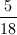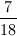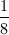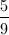Explanation:

There are 36 possible outcomes (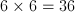). 10 out of the 36 outcomes are greater than 8: (6 and 3)(6 and 4)(6 and 5)(6 and 6)(5 and 4)(5 and 5)(5 and 6)(4 and 5)(4 and 6)(3 and 6).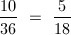Example Question #2 : Probability

Among a group of 300 people, 15% play soccer, 21% play baseball, and 9% play both soccer and baseball. If one person is randomly selected, what is the probability that the person selected will be one who plays baseball but NOT soccer?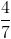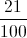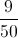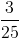Explanation:

Since there are 300 people,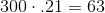people play baseball and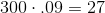of those people play both baseball and soccer. Therefore, there are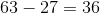people who play baseball but not soccer.

Probability: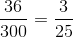Example Question #1 : Calculating Probability

If a die is rolled twice, what is the probability that it will land on either 2 or an odd number both times?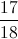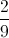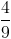Explanation:

probability on one roll: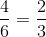for both times=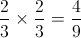Example Question #1 : Probability

What is the probability of sequentially drawing 3 aces from a deck or regular playing cards when the selected cards are not replaced?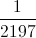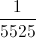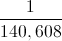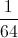Explanation:

The probability of drawing an ace first is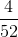or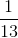.

Assuming an ace is the first card selected, the probability of selecting another ace is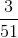or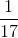.

For the third card, the probability is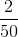or.

To calculate the probability of all 3 events happening, you must multiply the probabilities: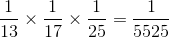Example Question #5 : Probability

How many even four-digit numbers larger than 4999 can be formed from the numbers 2, 4, 5, and 7 if each number can be used more than once?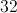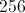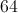Explanation:

Since the number must be larger than 4999, the thousand’s digit has to be 5 or 7. We are also told that the number must be even. Thus, the unit’s digit must be 2 or 4. The middle digits can by any of the numbers 2,4,5, or 7. Therefore, we have a total of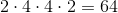possibilities.

Example Question #6 : Probability

What is the probability of rolling an even number on a standard dice?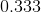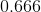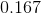Explanation:

A standard dice has 6 faces numbered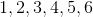.

There areeven numbers,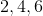, divided by the total number of faces: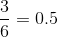Example Question #7 : Probability

Shawn is competing in an archery tournament. He gets to shoots three arrows at a target, and his best two shots count.

He hits the bullseye with 40% of his shots. What is the probability that he will hit the bullseye at least twice out of the three times?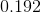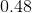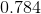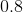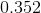Explanation:

There are three scenarios favorable to this event.

1: He hits a bullseye with his first two shots; the third shot doesn't matter.

The probability of this happening is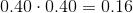2: He hits a bullseye with his first shot, misses with his second shot, and hits with his third shot.

The probability of this happening is3: He misses with his first shot and hits a bullseye with his other two shots.

The probability of this happening is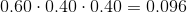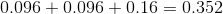Example Question #8 : Probability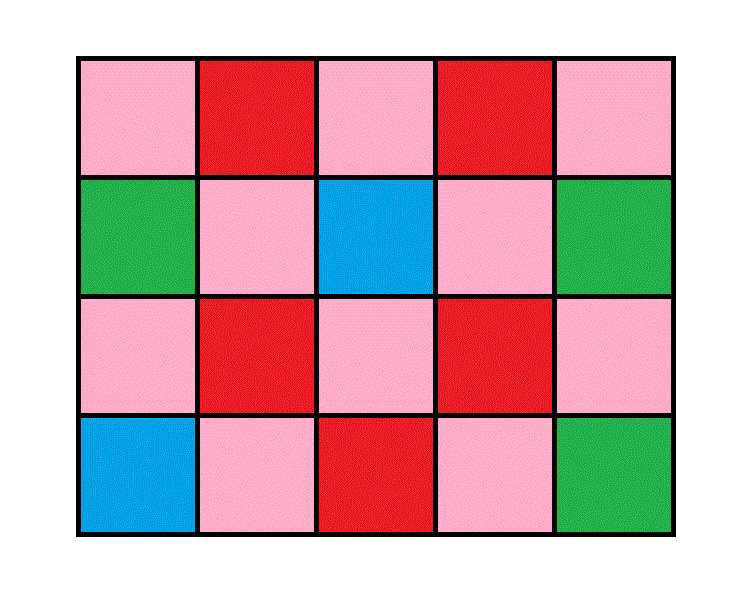A store uses the above target for a promotion. For each purchase, a customer gets to toss a dart at the target, and the outcome decides his prize. If he hits a pink region, he gets nothing; if he hits a red region, he gets a 10% discount on a future purchase; if he hits a green region, he gets a 20% discount; if he hits a blue region, he gets a 40% discount.

Assume a customer hits the target and no skill is involved. What are the odds against him getting a discount?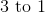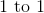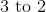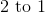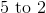Explanation:

The customer gets a discount if he does not hit a pink region. There are ten out of twenty ways to hit a pink region and ten to not hit one - this makes the odds 10 to 10, or, in  lowest terms, 1 to 1 against a discount.

Example Question #9 : Probability

It costs $10 to buy a ticket to a charity raffle in which three prizes are given - the grand prize is$3,000, the second prize is $1,000, and the third prize is$500. Assuming that all of 1,000 tickets are sold, what is the expected value of one ticket to someone who purchases it?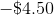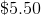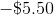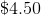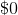Explanation:

If 1,000 tickets are sold at $10 apiece, then$10,000 will be raised. The prizes are $3,000,$1,000, and $500, so$4,500 will be given back, meaning that the 1,000 ticket purchasers will collectively lose \$5,500. This means that on the average, one ticket will be worth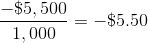This is the expected value of one ticket.

Example Question #10 : Probability

Daria has 5 plates: 2 are green, 1 is blue, 1 is red, and 1 is both green and blue. What is the probability that Daria randomly selects a plate that has blue OR green on it?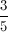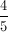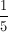Explanation:

The easiest way to solve this is by using the complement. Only one of the five plates is NOT blue or green. Soof the plates are NOT blue or green.  Therefore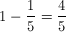of the plates are blue or green.

← Previous 1 3 4

Tired of practice problems?

Try live online GMAT prep today.